# cycloid

## roulette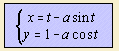The curve is formed by the locus of a point, attached to a circle (cycle -> cycloid), that rolls along a straight line1). In other words: the combination of a linear (term t) and a circular motion (terms sin t and cos t).
In a Whewell equation the curve can be written as s = sinφ.
The old Greek already knew with this curve.

The value of the parameter 'a' determines the starting point in relation to the circle:

• (ordinary) cycloid
The starting point is situated on the circle (a = 1).
When the starting point is not on the circle, the curve is called a trochoid:
• prolate cycloid (Fr. cycloďde allongée)
The starting point is situated outside the circle (a > 1).
• curtate cycloid (Fr. cycloďde raccourcie)
The starting point is situated inside the circle (a < 1).

When you have a steady hand, you can make your own cycloid on a blackboard, combining a linear and a circular motion.

When a cycloid rolls over a line, the path of the center is an ellipse. For the ordinary cycloid, the result is a circle.

## (ordinary) cycloid

In Holland we use for this curve also the name "wheel line" 2), being to the track followed by a point on a cycling wheel.

The curve has two remarkable qualities: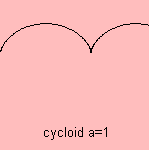The first quality is that the cycloid is the brachistochrone 3), that is the curve between two points in a vertical plane, along which a bead needs the shortest time to travel 4). Galilei (who gave the curve its name in 1699) stated in 1638 (falsely) that the brachistochrone has to be the arc of a circle. And in June 1696 Johann Bernoulli challenged his brother Jakob Bernoulli - the both were rivals - to solve the problem. December 1696 Johann 5) repeated his challenge in the 'Acta eruditorum', asking to send solutions before Easter 1697. Besides Johann and Jakob also Leibniz, Newton, and de L'Hôpital solved the problem.
This is one of the first variational problems, to be studied.
As a matter of fact, this curve is the opposite (mirroring in the x-axis) of the shown curve. Without any formula, it can be understood that along this curve the path is faster than along a straight line. For a cycloid the resulting component of the gravity is larger, and so the acceleration and speed, immediately after the start. It is also easy to verify experimentally that the way along a straight line takes more time.
This knowledge can used while skiing: it is faster choosing a way down so that you gain speed, than to avoid the slopes.

The second quality is that the cycloid is the tautochrone (sometimes called: isochrone) 6). This means that a bead along the curve, needs the same time to get down, independent from the starting point. Wonderful! It was Christiaan Huygens who discovered this fact, in 1659. In his treatise 'Horologium Oscilatorium' (1673) he designs a clock with a pendulum with variable length. The pendulum moves between two cheeks, both having the form of a cycloid. Swaying to the outer side, the pendulum shortens. Huygens used the insight that the involute of a cycloid is the same cycloid (of course also valid for the evolute).
By this construction the irregularity in a normal pendulum was compensated. For a normal pendulum then, the time of oscillation is only in a first approximation independent of the position aside 7). Huygens was quite impressed, he wrote above the prove: 'magna nec ingenijs investigata priorum', to be translated as: 'this is something great, and never before investigated by a genius'.
In his experiments Huygens used also the involute of a circle in his pendulum clock to approximate the cycloid path.

However, use of the tautochrone principle in designing pendulum clocks posed too many mechanical problems, to make it common.
The tautochrone quality can also be read in Moby Dick, the book from Herman Melville, in a treatise about a kettle to boil whale oil.

Some interesting properties of the cycloid are the following:

Cusa was the first to study the curve in modern times, when trying to find the circle's area. Mersenne (1599) gave the first proper definition of the cycloid, he tried to find the area under the curve but failed. He posed the question to Roberval, who solved it in 1634. Later, Torricelli found the curve's area, independently.
Descartes found how to draw a tangent to the cycloid, he challenged Roberval to find the solution, Roberval failed, but Fermat succeeded. Also Viviani found the tangent.

In August 1658 Pascal published a challenge, offering two prizes, under the name of Amos Dettonville. He posed 9 questions on the cycloid, asking for the area and the center of gravity of its segment.
It is said that for Pascal studying the curve was a good diversion from his severe toothache.
Wallis and Lalouére entered, both were not successful. Sluze, Ricci, Huygens, Wren and Fermat did not enter the competition, but all wrote their solution to Pascal. On October 10 1658, Pascal published his own solutions, together with an extension of Wren's result.
The name of the paper was: 'Histoire de la Roulette, appelé autrement Trochoide ou Cycloide'.

Desargues proposed teeth for gear wheels, in the form of a cycloid (about 1635).

Jakob and Johann Bernoulli showed (1692) that the cycloid is the catacaustic of a circle, where light rays come from the circumference.
A cycloid arch, with rays perpendicular to the x-axis, results in two cycloid arches.

So the cycloid was very popular among 17th century mathematicians. That's why later the curve has been given the names of quarrel curve, Helen of Geometers, and apple of discord9).

In the Piano Museum in Hopkinton10) one finds a piano, whose back edge has the form of a cycloid. The maker, Henry Lindeman, named the instrument 'the Cycloid Grand', in the late 1800s.
But seen from the upside you see that its form differs from a real cycloid: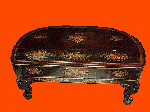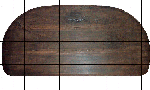Gear wheels have a cycloid form, they can be approximated by a series of circular arcs. Also numeric tables can be used, as George Grant's Odontograph, which is also the name for an instrument for laying off the outlines of the teeth of the gear wheels.

The curve is a point-roulette.

## trochoid11)

Now the point being followed is not lying on the circle. When the point lays outside the circle, the curve is called a prolate cycloid (or extended cycloid). When the point lays inside the rolling circle, the curve is called a curtate cycloid (or contracted cycloid). The latter curve is followed by the valve of a bike. That's where the name valve curve for the cycloid is from.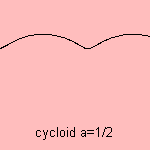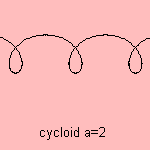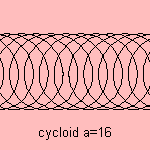The first to study the curve were Dürer (1525) and Römer (1674).

With a Voith-Schneider Propeller (VSP), first tested in 1927, a ship is able to maneuver precisely, and to move aside. The propellers rotate around an axis, vertical to the motion, so that they follow the path of a cycloid. The position of the blades determine the direction of the vessel, and it is based on the same principle of a fish's fin action.
The propeller is called a cycloidal or trochoidal propeller.

notes

1) Let there be a circle with center (0,R) and a point  (p, 0) as starting point to roll. Then the coordinates of the cycloid, as function of the rolled angle t are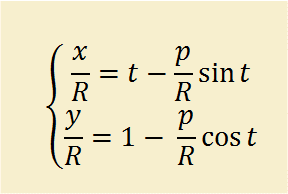2) In Dutch: radlijn

3) Brakhisto (Gr.) or brachus (Lat.) = short, chronos (Gr.) = time

4) At height y the bead becomes a velocity √gy, so that minimizing the travel time means minimizing the integral.
Solving this equation leads via differential equation y (1 + y'2) = c to the cycloid.

5) In that period professor in mathematics in Groningen, Holland

6) Tauto = equal, chronos = time: the curve to be followed in equal time.

7) The correct relation is given by a complete elliptical integral of the first kind.

8) In English: A history on the roulette, also named trochoid or cycloid.

9) Helen and the apple of discord refer to the Trojan war.
Dutch for quarrel curve: kibbelkromme.

10) Hopkinton, Mass., about 1/2 hr. west of Boston, see The Piano Museum website.

11) Trochus (Lat.) = hoop.
Sometimes the meaning of cycloid and trochoid is interchanged: trochoid for the general case, cycloid only for the situation that the starting point is lying on the circle.